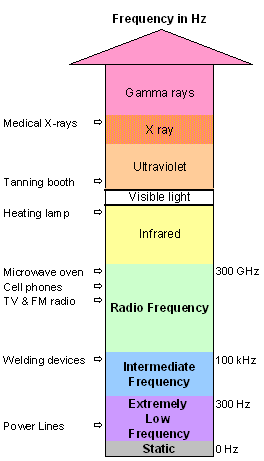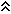ABC - DEF - GHI - JKL - MNO - PQRS - TUV - WXYZLanguages:English [en]Frequency Similar term(s): Hertz, f. Definition: Frequency is the measurement of the number of times that a repeated event occurs per unit of time. The frequency of wave-like patterns including sound, electromagnetic waves (such as radio or light), electrical signals, or other waves, expresses the number of cycles of the repetitive waveform per second. In SI units, the result is measured in Hertz (Hz), named after the German physicist, Heinrich Rudolf Hertz. 1 Hz means one cycle (or wave) per second. Frequency has an inverse relationship to the concept of wavelength (the distance between two peeks) such that the frequency is equal to the velocity divided by the wavelength. Source: GreenFacts More: Frequency ranges for different types and sources of electromagnetic fieldsSource: GreenFacts ABC - DEF - GHI - JKL - MNO - PQRS - TUV - WXYZTop